Next: Attention Up: The ACTION Network Previous: The ACTION Network

## Active Memory

In order to obtain some idea of the properties of the solution of equations (27) the first approximation to them will be that of linearisation. Absorbing the derivatives of the output functions into the strengths of the connection matrices, and dropping the input J and the lateral connection matrix, the equations may be reduced to the single equation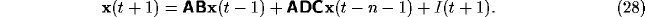For the case that I is purely an initiator of activity, the growth of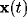is exponential in time, with behaviour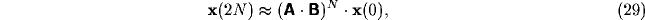and so has time constant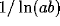, where a and b are the diagonal elements of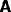and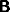(when they are diagonal). In the case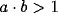the behaviour (29) will be of exponential increase, until the non-linearity in the neuronal responses, especially that of saturation, becomes important. For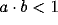, the activity would initially be expected to die away. In that case, it is necessary to take more careful account of the basal ganglia contribution. Then, again in the diagonal case, and reducing to the largest eigenvalue of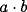, for the behaviour ofas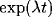, there results the solution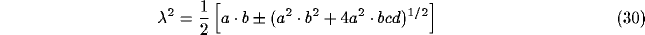In the case that all of a, b, c and d are positive, which seems supported by the neurobiology, then it is still possible to have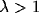in (30) even though. This is so for any value ofprovided that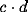is large enough.

Given that it is always possible to have an increasing activity on the frontal cortex, for suitably large basal ganglia to thalamus connection weights, it is then possible to see that this activity will saturate when the non-linear response function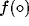in equation (27) is used. To see this, it is only necessary to include the non-linearity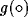of the thalamic output. Equation (27) then becomes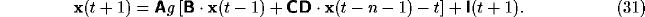The asymptotic value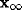will therefore satisfy the equation (dropping the external input)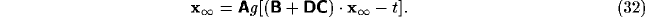This has a bounded solution, for any connection matrix. The general form of the temporal development of the activities of the modules will thus, for large enough strength of connectivity from basal ganglia to thalamus (as noted above), be of an initial exponential rise of activity, followed by saturation for a period depending on the external input. If that latter acts in an inhibitory manner at a certain time value, then it is possible to see that this will cause the activity of equation (32) to die away. This would agree with the general form of the experimental activities shown, for example, on the left hand column of Figure 5 of Zipser .

The presence of more than one attractor is clear from the relation of (31) to a BAM [24,30], to which it is identical when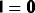,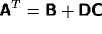. More generally a number of attractors will be expected, with noise causing transitions between them [25,53].

The training necessary to achieve such active memory behaviour is obtainable through simple Hebbian learning on the basal ganglia connection weights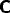and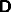, since as these increase they will enable the lifetime of the neuronal activity of the cortical nodes to increase, according to equation (30). This has already been investigated in , to which the reader is referred.

An important feature of active memory is whether or not the ACTION net acts as a flip-flop for non-zero input. Thus the simplest ACTION net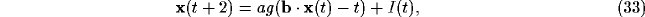in the limit thatis the Heaviside step function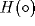, is turned on permanently by an input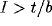, but only transiently for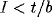. Replacingby a smooth sigmoid function in (33) will then lead to a smoothly increasing and saturating response to a large enough input, but a transient decaying response to a smaller one. The first of these two possibilities are observed in the cells of Figure 1 of Zipser et al. , and in the growing population vector in Figure 11 of Georgopoulos et al. ; the latter is presented in Fuster .

It is possible to model the growth of neuronal activity in the Georgopoulos et al. results as follows. For N neurons, each with optimal angular direction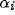for the i'th neuron, let that cell have input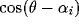arising from some directional input with information that it involves the vector (angular) direction. For the linear case, with t=0 in (32) (and diagonal connection matrices), the asymptotic activity of each ACTION cortical neuron becomes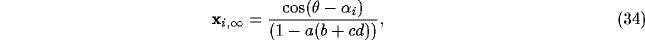(where it is assumed that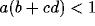). Then the population vector output points along the desired direction, since this vector has value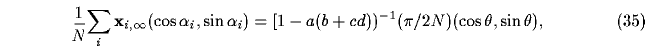where only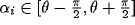has been used to have a positive output from the neurons in (34), and the sum over random directions i in that interval for the cross terms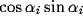may be shown to be zero for large enough N. The time constant to rise to saturation of about 200 msec  would be expected to be obtainable for a suitable range of connection weights and thresholds.Next: Attention Up: The ACTION Network Previous: The ACTION Network